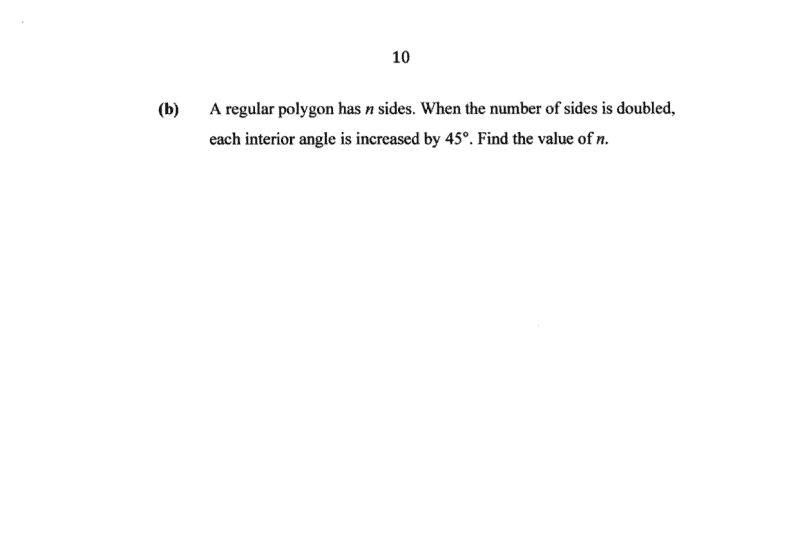# Questionpls help

The formula for the sum of angles within a polygon is (n-2) x 180

Since this is a regular polygon, all the sides are equal and therefore each of its angles is equal/the same.

Therefore the angle of each side is: [(n-2 x 180)]/n = 180 – (360/n) …. (1)

When n is doubled, the angle of each side is now: 180-(360/2n) = 180-(180/n) …. (2)

When n is doubled, each interior angle is 45 degrees more.

Therefore we will form this equation, (2) – (1) = 45

[180-(180/n)] – [180-(360/n))] = 45

180 – (180/n) – 180 + (360/n) = 45

180/n = 45

45n =180

n=4

We can double-check if this is correct by testing it out.
If this is really a 4 sides figure, aka a square, each side is 90 degrees.

When it becomes an 8 sided figure, octagon, each side is 135 degrees.

Therefore there is really a 45-degree increase and n is indeed 4.

0 Replies 1 Like ✔Accepted Answer